# Nickzom Calculator Calculates the Total Time of Flight and Time of Flight at Maximum Height in Projectile (Physics)

I am not hear to start explaining to you the meaning and application of time of flight. I believe you are here to know how the calculator encyclopedia calculates these parameters.

Nickzom Calculator requires three parameters to get the answer for time of flight and these parameters are:

• Acceleration due to Gravity
• Initial Velocity
• Angle of Projection

Let’s first get the workings and answer for time of flight at maximum height where the acceleration due to gravity is 10 m/s2, initial velocity is 20 m/s and angle of projection is 30°.

First and Foremost, Select Physics from the home page and click on Open Section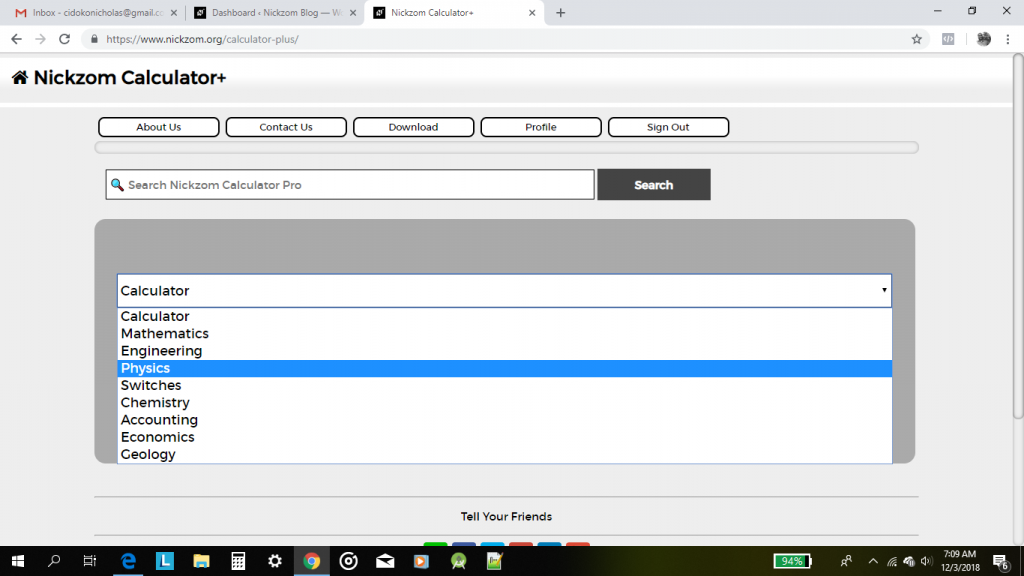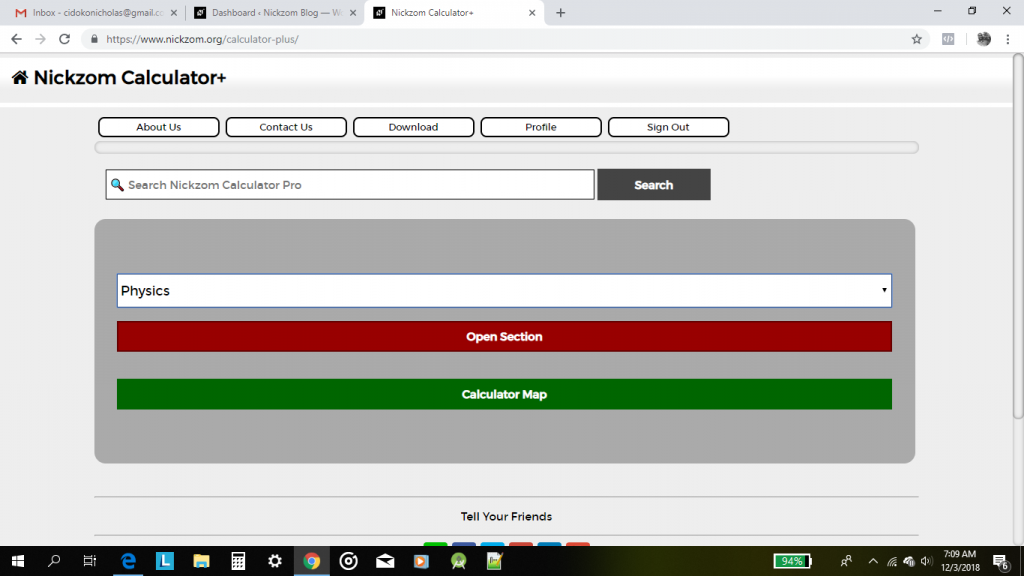In the Physics section, Click on Projectile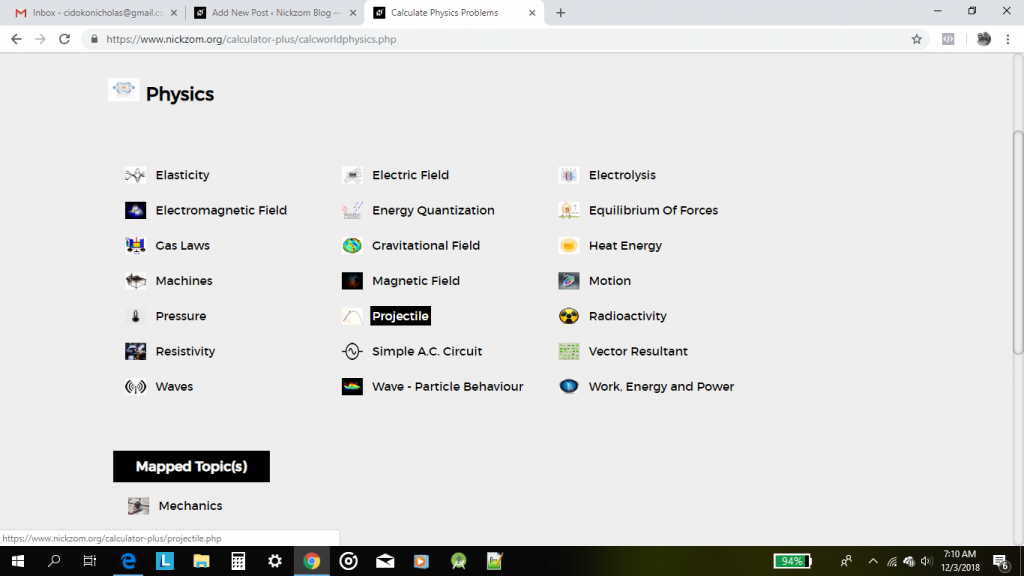Now, click on Time of Flight at Maximum HeightAn interface for you to enter the parameters to get the answer for time of flight at Maximum height is displayed for you.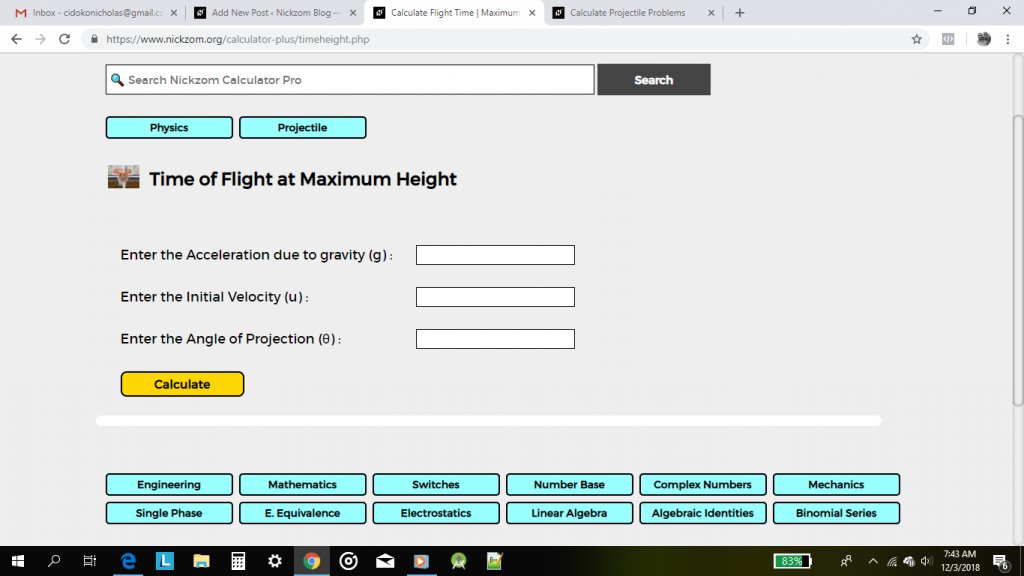Here is the part where you need to enter the accurate values according to their parameters.

According to the problem we want Nickzom Calculator to solve stated above,

Acceleration due to Gravity: 10

Initial Velocity: 20

Angle of Projection: 30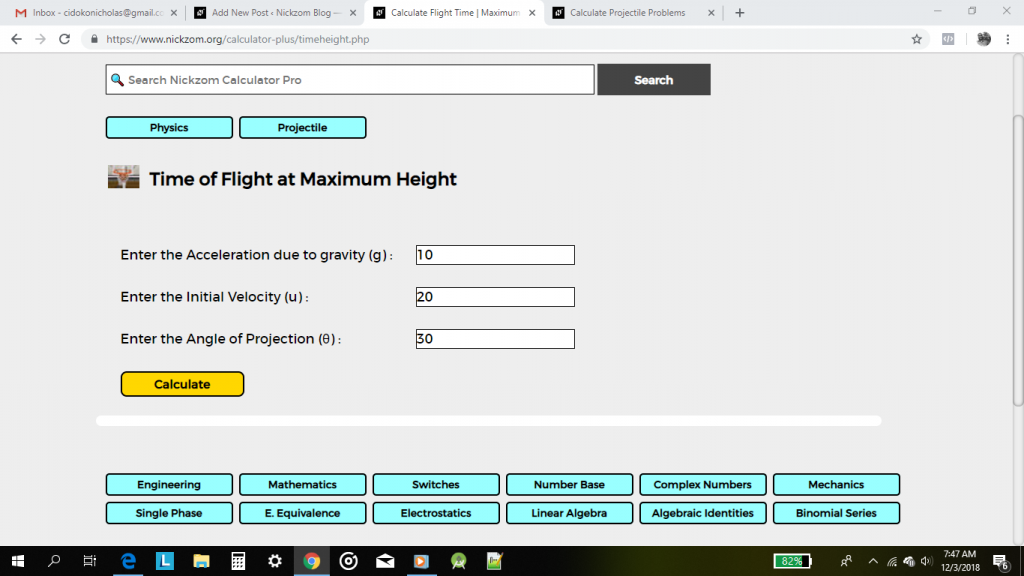Simply, click on the Calculate button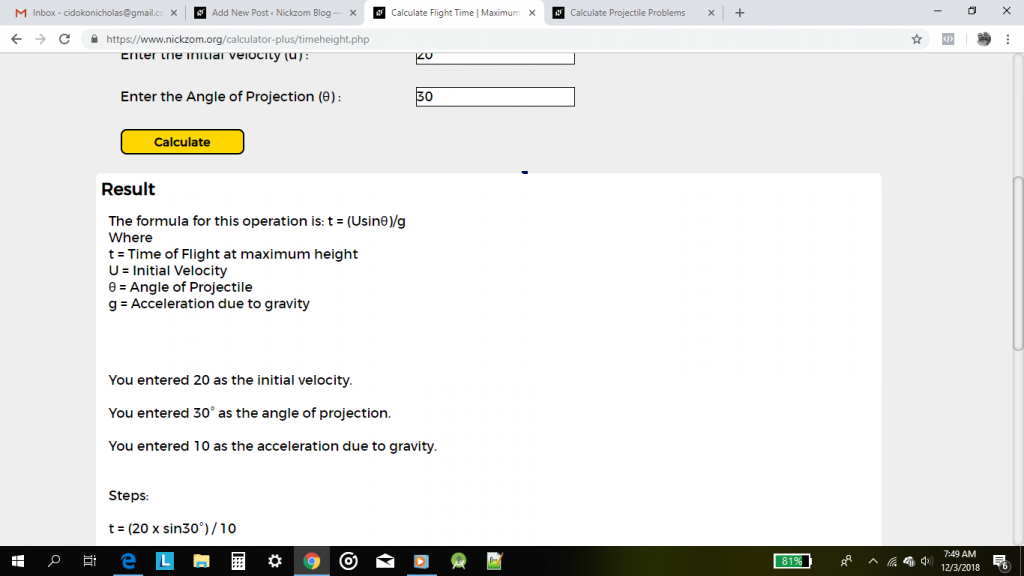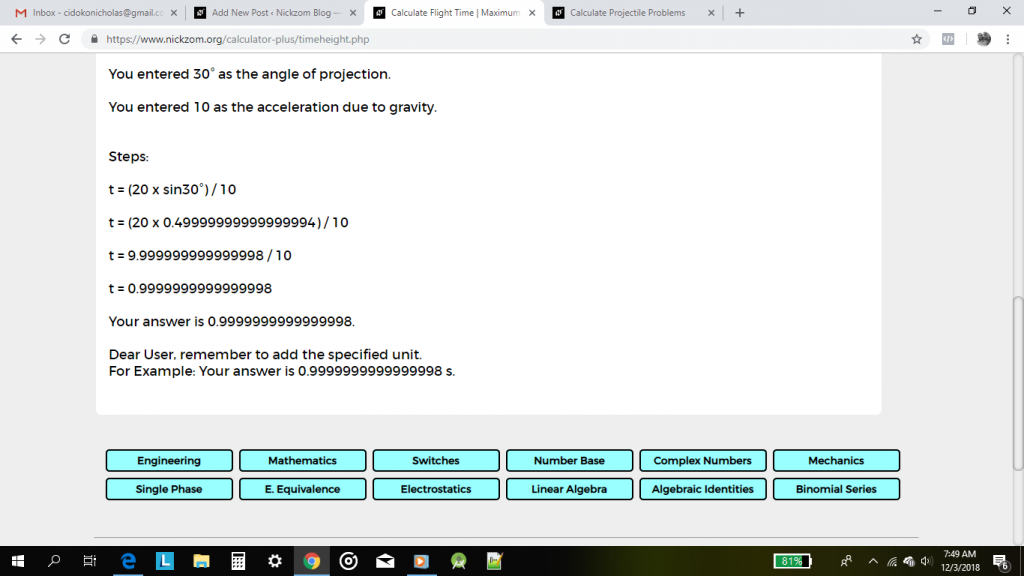Amazing Right? Nickzom Calculator displays the steps (workings), formula and answer.

Now, let’s work on Total Time of Flight. Using Nickzom Calculator get the answer for total time of flight where the acceleration due to gravity is 9.81 m/s2, initial velocity is 10 m/s and the angle of projection is 60°.

First, we navigate back to the Projectile Section, How you say? Scroll to the top of the page you are currently in. Then click on Projectile in the middle navigation bar. The screenshot below displays the one to click.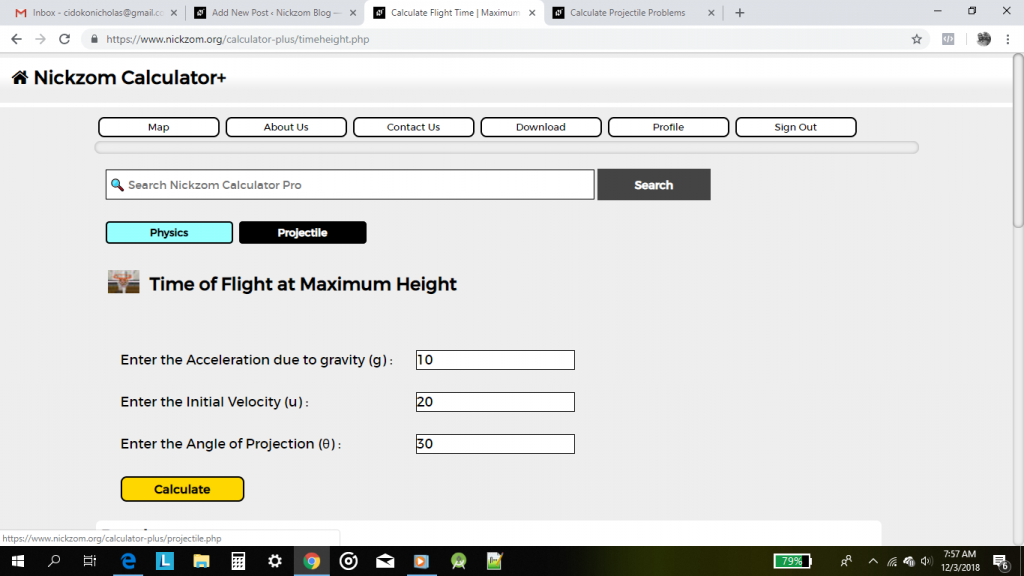On clicking on Projectile, you get redirected to the Projectile section. Now, click on Total Time of Flight.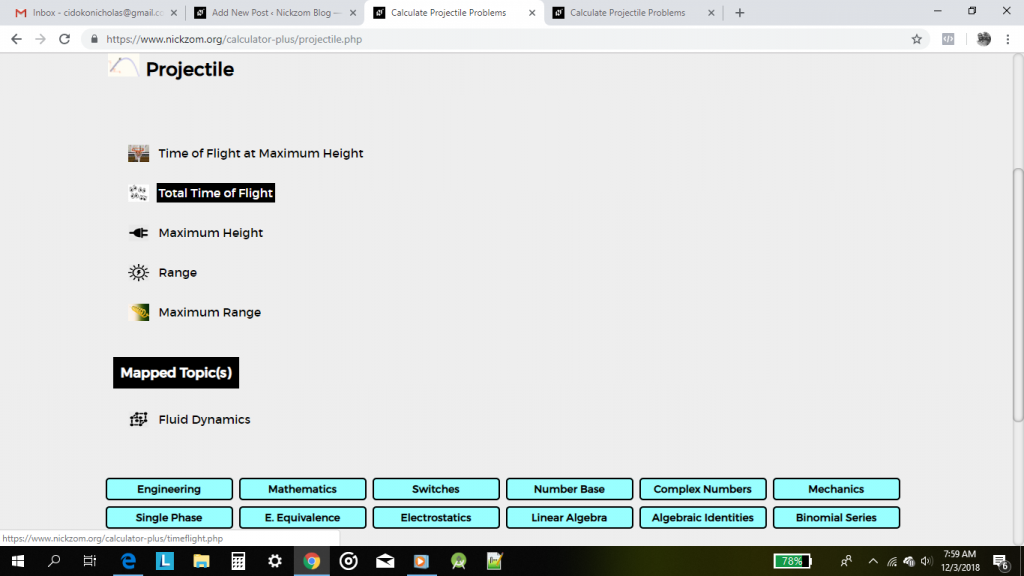On clicking displays this interface below, for you to enter the appropriate values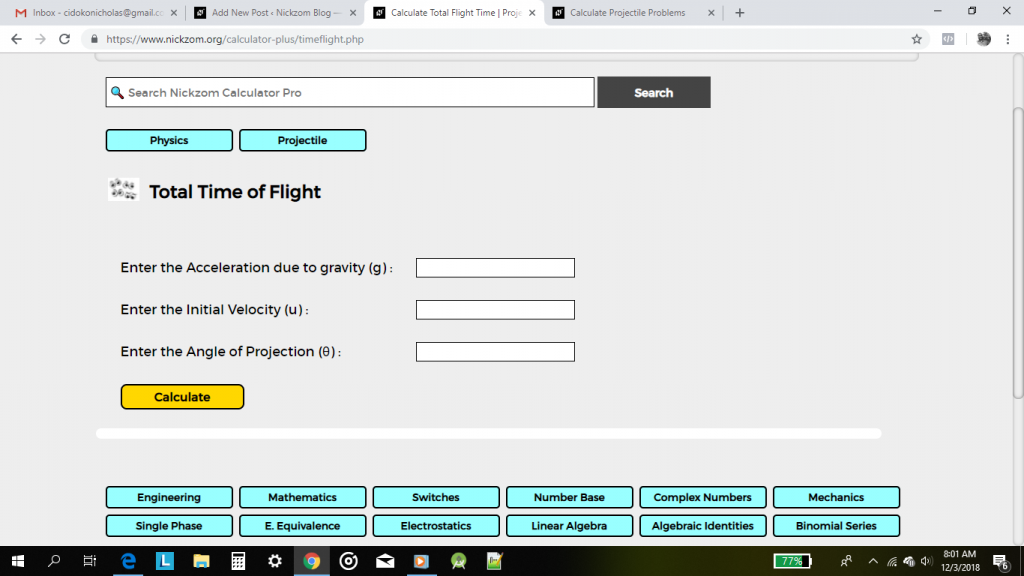Now enter the values according to the problem provided to get the accurate answer

Acceleration due to Gravity: 9.81

Initial Velocity: 10

Angle of Projection: 60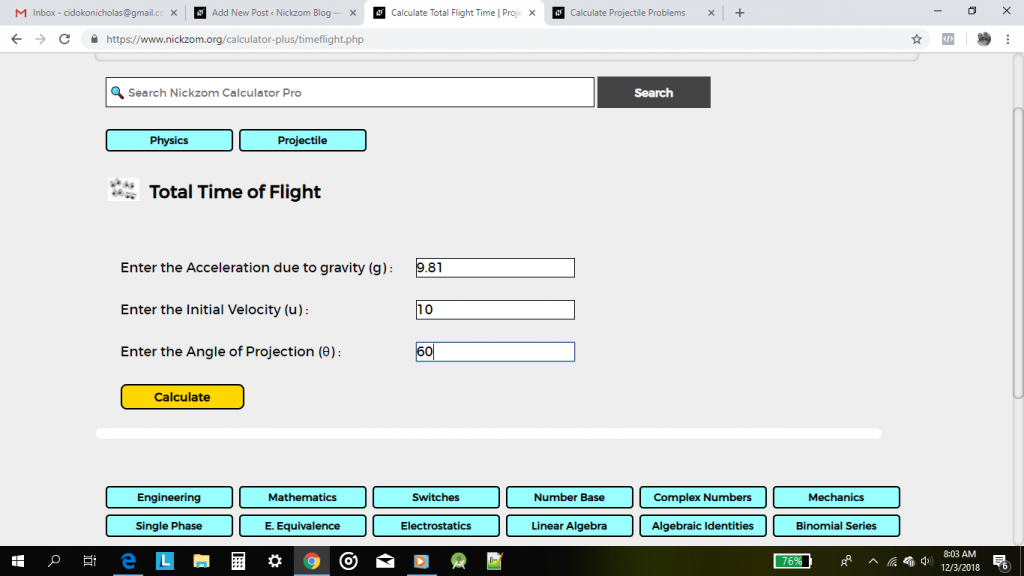Lastly, click on the Calculate button.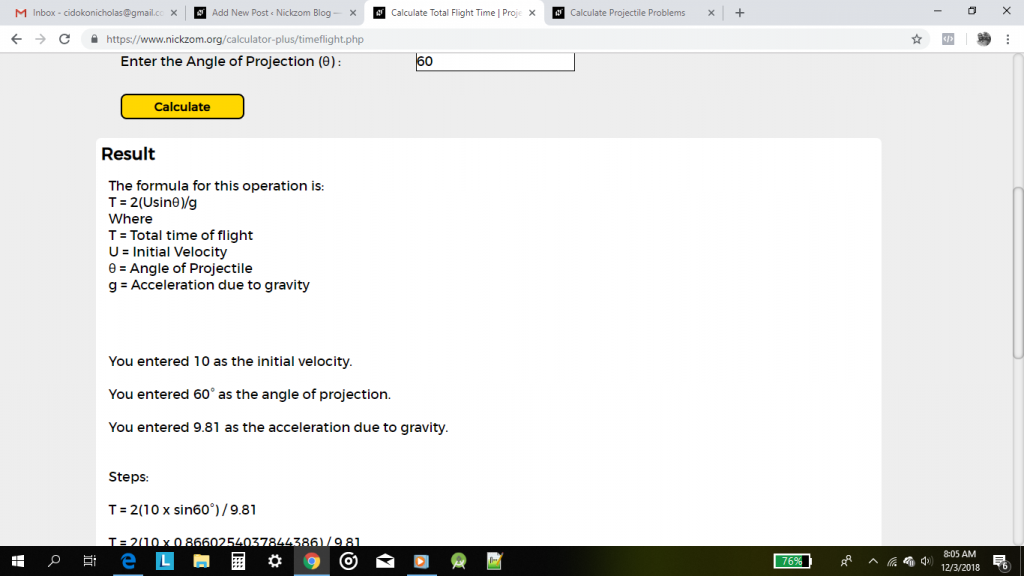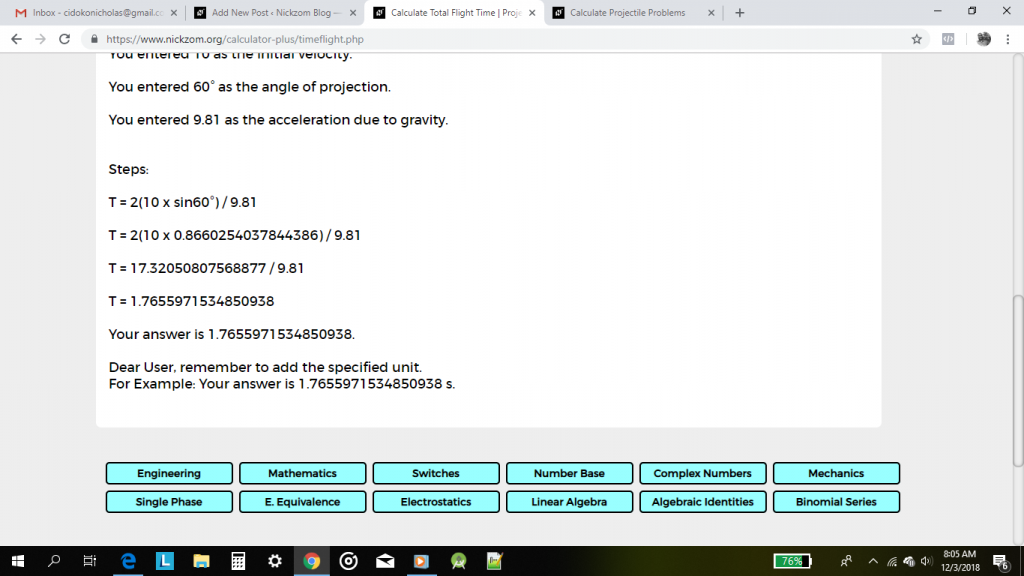You can access Nickzom Calculator (The Calculator Encyclopedia) via any of these channels: